﻿ Discovering Weight Knowledge Based on Linguistic Information Aggregation

# Full Text Article

## Discovering Weight Knowledge Based on Linguistic Information Aggregation

Received Date: December 22, 2020 Accepted Date: January 22, 2021 Published Date: December 27, 2020

doi: 10.17303/jcssd.2021.1.101

Citation: CHEN Shuguo (2021) Discovering Weight Knowledge Based on Linguistic Information Aggregation. J Comput Sci Software Dev 1: 1-8.

Weight knowledge is important in many fields in decision science. For example, in an artificial neural network (ANN), knowledge resides in the weights on links between neural neurons, based on which output is obtained from given inputs. Although a meaningful output of an ANN is of great use, implications of individual weights are often difficult to decipher. In the context of qualitative decision making, linguistic variables often express information that is about ordinal ranking rather than an exact quantity, and therefore the challenge lies in how to determine the weights conforming to the reality and making sense of the decision. In this work, we discriminate two kinds of weights, where one kind is related to the importance of attributes, and the other is related to aggregation characteristics. We propose a weight estimation method, in which these two kinds of weights are calculated simultaneously from a set of known cases that provide additional aggregation information. Using a simulated annealing algorithm, weights satisfying certain conditions can be obtained, and results for new cases can be aggregated thereafter. We find that the weights developed in our method are more explanatory than other approaches, such as the artificial neural network.

Keywords: Linguistic Information; Weights Aggregation, Weight Discovering, Simulated Annealing Algorithm

Aggregation of criteria functions to form an overall decision function is important in various fields in decision science. In the simplest cases such as when both outputs of the criterion functions and the overall decision function are numerical values, the knowledge lies in determining the criterion weights, by which one can get the overall grade by calculating a weighted average of all criteria grades. In more complex situations, the output can no longer be represented as a linear function of inputs. For example, when an artificial neural network (ANN) is trained by a set of instances with known results, the knowledge is embodied in weights on links between neural neurons.  However, the benefit of an ANN is achieved at a cost, which is the inherent inability to explain, in a comprehensible form, the process through which a decision or output is given.  To address this issue, some complementary techniques such as extracting rules from ANN have been studied (see [1, 8] for example).

Uncertainties in inputs and outputs also bring complexity. [21, 22] The Fuzzy Set theory, proposed and developed by Zadeh  is often used to deal with the situation where the assessment information is stated in linguistic terms. Membership functions can then be defined to denote the degree of truthfulness of the proposition. Evidence theory, of Shafer , is also useful in aggregating information when uncertainty is attributable to ignorance, instead of fuzziness . Because of the difficulties in defining consensus membership function and approximation of the ultimate irregular fuzzy set, a method that directly operates on linguistic variables has been proposed in [9-11]. The method is based on the concepts of ordered weighted averaging (OWA) operators developed by Yager  and convex combination of linguistic labels defined by Degani and Bortolan. 

Aggregating linguistic information has large potential in practice.  For example, when assessing the attributes of cars such as acceleration, braking, handling, ride quality and powertrain, customers often use linguistic terms, or linguistic variables, to describe their opinions. Therefore, how these individual attribute assessments can lead to an overall ranking becomes a complicated problem. Although linguistic ordered weighted averaging (LOWA) operator  can provide an aggregation mechanism, it assumes that inputs are equally important. Therefore, it becomes difficult to develop the weights accordingly, which implicitly determine the degree of “anding” and “oring” in the aggregation.

In this work, we develop a method that extends the LOWA operation by removing the “equal importance” assumption in the inputs. The knowledge about the aggregation mechanism and importance of each input variable can be calculated from a set of known cases with additional aggregation information.

This paper is structured as follows. In Section 2, the problem is briefly reviewed. The LOWA operator and the weights in different means are discussed in Section 3. In Section 4, we propose an operator allowing for two kinds of weights, and present how it can be used to aggregate linguistic information based on mining known behavior cases. Numerical examples and related computation are illustrated in Section 5. Finally, the conclusion together with some discussion are given in Section 6.

Let A1 , A2 ,..., An be n criteria in a multi-criteria problem. X is the set of alternatives. For a proposed alternative x € X , Aj(x) indicates the performance of x according to Criterion Aj. The assessment of an alternative against other criterion or itself may be qualitative. Our problem is to develop a proper method to get the overall assessment of x from the set of {Aj (x) | j =1,...,n}.

A number of alternatives with known results are available, which act as a series of cases provided for case-based reasoning. There may also be other form of knowledge on hand such as comparative knowledge of two criteria’s importance, etc.

When the performance of alternatives can be assessed quantitatively, an ANN can be expediently trained to learn the aggregation process. However, people still need the knowledge on weight allocation in the network to help comprehend and make sense out of the decision.

As Yager pointed out , when all criteria are equally important, the aggregation structure can be viewed as between two extremes, “anding” and “oring”. At one extreme, the overall result is good only if all the criteria are satisfied. Hence the output is the minimum of all the inputs. At the other extreme, the overall result is good as long as at least one of the criteria is satisfied. The output can then be calculated by maximizing all the inputs.

More generally, if the domain of each Aj is [0,1], and all criteria are equally important, then the aggregation process can be expressed by an OWA operator F with weighting vector v = (v1, v2, ….vn)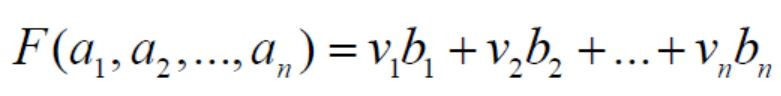where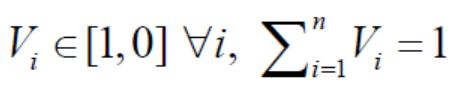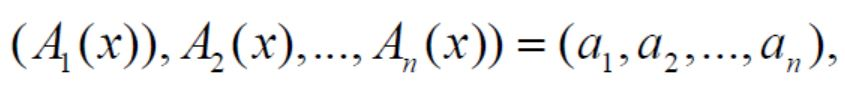bi is the i th largest element in the collection {a1 ,a2 ,...,an }.

Vector v can determine the “andness” or “orness” of the aggregation. v = (1,0, … ,0) corresponds to the pure “or” operator, while v = (0,0, … ,1)corresponds to the pure“and” operator.

In many real circumstances, it is more desirable that Aj (x) s present a linguistic value. For example, their domain can be a linguistic expression set S = {si |i ∈{0,...,T}}, used to provide performance value about alternatives according to various criteria. For instance, S can be the set of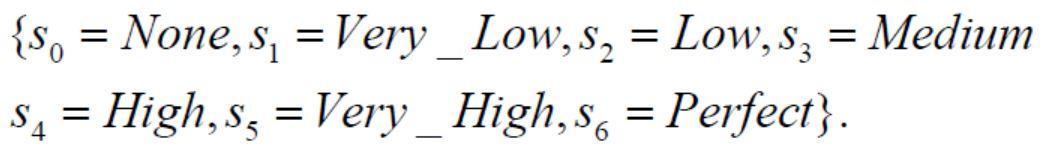If the term set has the following characteristics:

• The set is ordered: si ≥ sj if i ≥ j ;
• There is the negation operator: Neg (si) = sj such that j = T − i

An aggregation operator, LOWA φ can be defined to compute directly from linguistic labels . For the label set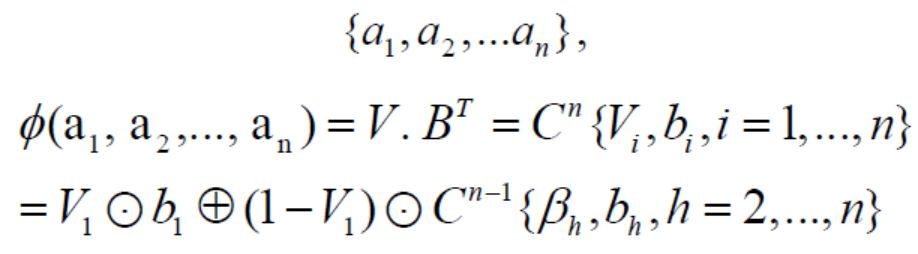where, v and B = {b1,...,bn } are as before,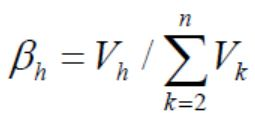, h = 2 ,...,n, and Cm is the convex combination of m labels and if m = 2 ,C2 is defined as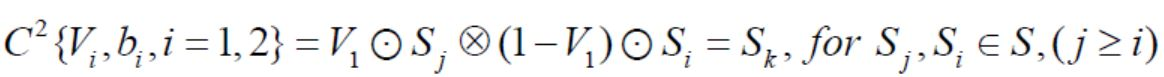such that k = min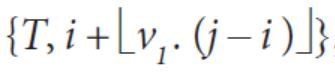, where b1 = sj, b2 = si.

If vj = 1 and vi = 0 with i ≠ j,∀i, then the convex combination is defined as: Cm {v1, b1, i = 1,...,m} = bj

Therefore, it is easy to see that how to calculate the weighting vector of LOWA operator v is a basic question to be resolved.

On the other hand, weights in LOWA operators determine the structure of aggregation, so can be viewed as aggregation weights. If the attributes or criteria are not really of equal importance, another kind of weights should be taken into account. Issues of weighted aggregation operators have been studied in [6, 7, 9-12, 16, 19, 23, 25]. In the following section, how the importance weight vector w of criteria can be integrated into the aggregation process, and how they can be identified from a set of cases are studied.

The weights bound with attributes are more intuitive for assessing alternatives. Most of the information aggregation methodologies in literature presume the weights are assigned in advance, but in reality, it is not easy for the decision- maker to give a certain and consistent weight vector. Some improvement ideas include attribute weights as fuzzy numbers (e.g. [2, 24]) or linguistic variables (e.g. [4, 18]).

In our method these weights are regarded as interior quantitative variables implied in the aggregation results. They are initially unknown, but can be defined and calculated, and used to aggregate information thereafter.

Let the importance weight vector of criteria be w = (w1, w2,..., wn ):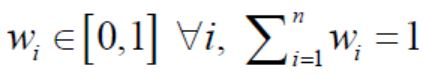.

Considering both the aggregation weights and the attributed weights, for some alternative x : ( A1 ( x), A2 ( x),..., An ( x)) = (a1, a2,..., an ), an aggregation operator φ can be defined as: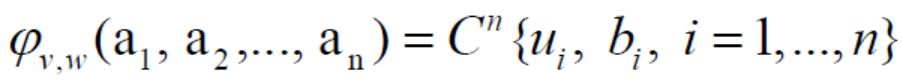where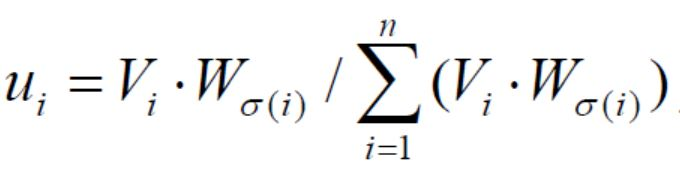, i = 1,...,n , σ is a permutation over {1,2,…,n such that (b1, b2,..., bn ) = (aσ(1), aσ( 2),..., aσ( n ) ).

For the operator φv, w (we can call it LOW2A), we have simple properties as follows.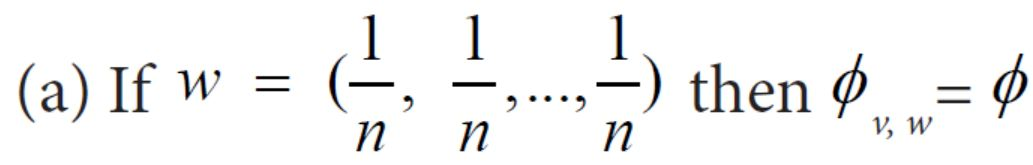b) If wi = 1 for some i ∈ {1,2,...,n} and v1 ≠ 0 for all i, then φv, w(a1, a 2,..., an ) = ai Because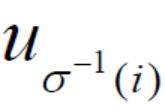= 1, and uj = 0 with j ≠ σ-1(i), ∀j, we have φv, w (a1, a 2,..., an ) =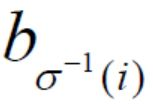= ai.

c) If wi = 0 for some i ∈ {1,2,...,n}, then φv, w(a1, a 2,..., an ) is independent of ai.

This is obvious from the fact that= 0 and the definition of the convex combination Cm.

The LOW2A operator φv, w can be illustrated by a simple example.

Assume the aggregation problem involves 4 criteria. v = (0.25,0.25,0.25,0.25), which implies both the “orness” and the “andness” of aggregation are 0.5. An alternative x has criteria assessments (s0, s3, s2, s4 ).

If four criteria are equally important, then the aggregation result for x by ordinary LOWA operator will be φ = s3

However, if the criteria weight vector is given, for instance, w = (0.2,0.3,0.4,0.1), then one can calculate the composite weight u = (0.1,0.3,0.4,0.2), and φ = s2. This comes from the fact that importance of the third largest element has been improved.

Weight discovering model

By means of the LOW2A φv, w operator defined in 4.1, the aggregation mechanism can be mapped to configuration of the weights v and w. Taking v1,..., v2, w1,..., wn as variables, according to the cases and information given, a mathematical programming can be built to estimate the weights.

a) Each alternative case (a1, a 2,..., an ) with known result Sk can be transformed into a constraint equation.

φv, w (a1, a 2,..., an ) = Sk

b) The information about the comparison of criteria importance should be expressed by equation or inequality in weight variables.

c) The objective function can be maximizing or minimizing the dispersion of v , or w , or both, where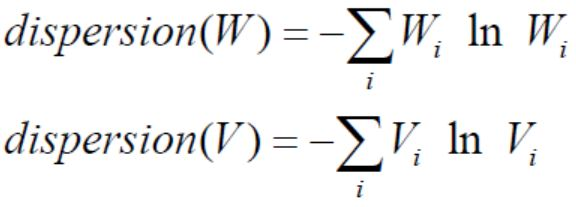Model and numerical examples

In this section, we make use of an example to demonstrate how the approach can be applied.

For a collection of alternatives, each can be assessed based on a set of attributes, to get an overall assessment. In group decision making context, information aggregation can refer to producing a comprehensive evaluation from a group of decision makers’ evaluations. In this example, we have four alternatives, and for each one, four linguistic assessments can be obtained from 4 experts according to their own specialties. We are interested in finding aggregation knowledge in terms of aggregation weights and expert weights.

Suppose we have a set of m alternatives, whose assessments results are according to the experts and their actual performance ranks are known. Let the alternative set be {c1, c2, c3, c4 }. For each alternativeci , we have its attribute linguistic evaluation A(ci ), and actual total performance (ci ), as follows.

a) For c1 :{a11 = s1, a21 = s5, a31 = s4, a41 = s6}, actual performance is s5.

b) For c2 :{a12 = s1, a22 = s3, a32 = s3, a42 = s1}, actual performance is s3.

c) For c3 :{a13 = s4, a23 = s5, a33 = s3, a43 = s2}, actual performance is s2.

d) For c4 :{a14 = s1, a24 = s6, a34 = s1, a44 = s0}, actual performance is s1.

Then, a mathematical programming model for minimizing the sum of dispersion of v and of w can be described as: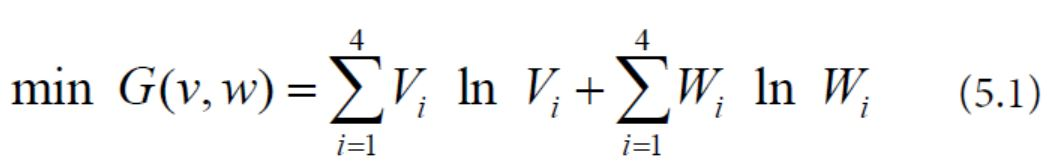S. t.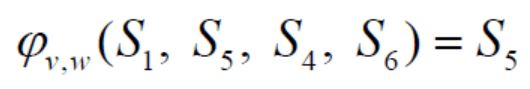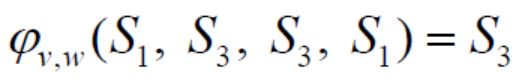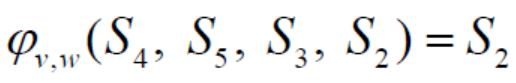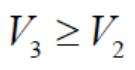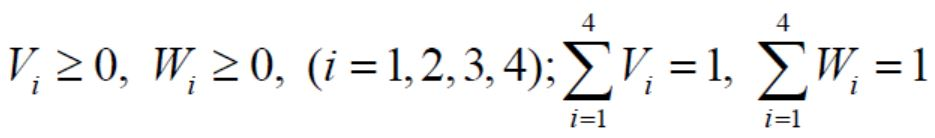A simulated annealing solution approach

As we can see, the model is formulated as a nonlinear mathematical programming model, which is difficult to solve to obtain exact optimal solutions. Therefore, in this paper, we propose a meta-heuristic approach, simulated annealing , to generate feasible solutions and then optimize heuristically.

The simulated annealing algorithm minimizes the non-linear objective function G(v, w) (Eq.(5.1)) from an initial vector based on a series of random moves. A group of parameters determine how a move is generated, and whether it is accepted, and how many times moves occur before temperature gets changed. Among the parameters, p defines the probability used in the generation of a random move. γ determines the initial temperature T0 as γF(X0) where X0 is the initial solution. r refers to the number of random moves before temperature is changed. β specifies the temperature reduction rate, where 0 < β < 1. The simulated annealing algorithm is illustrated as follows.

1) Select an initial solution X0 . Set the temperature T0 = γF(X0).

2) Repeat the following steps r times:

a) Generate a random move. Each element in the vector has probability p unchanged and probability 1-p replaced by a new random value.
b) Calculate the objective value of the new vector X.
c) Update the current configuration to the new vector X if a better objective value is found.
d) Otherwise, let ΔF denote the increase in the value of the objective function. Update the current configuration to the new vector X with probability p1 = exp(ΔF / T). Keep the current configuration with probability 1 − p1.

3) Reduce the temperature by multiplying the time reduction parameter β.

4) Terminate when an optimum value is obtained or the objective value cannot get improved after certain number of temperature changes.

In order to find a feasible solution weights, we set the objective function as follows.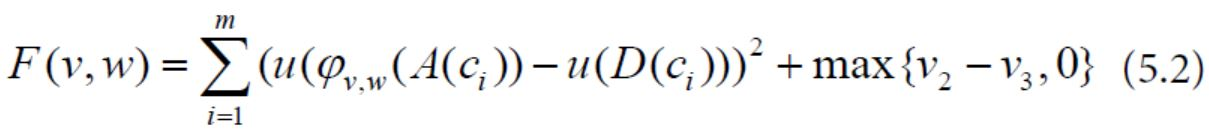In Equation (5.2), u is the utility for evaluation rank, which is set as u(si) = i for symmetric and even linguistic term set. For asymmetric terms set, u is set according to .

We notice in a standard simulated annealing algorithm, a random move on the weight element wi may dramatically change the whole weight vector (w1, w2,...,wn), and therefore we apply the following customized procedure to keep some characteristics of the weight vector unchanged when implementing a random move. We select a non-zero wj , say, the first one greater than 0.2 in our example, and keep each wi / wj for i ≠ j unchanged with probability p and change it to max {wi / wj + uniform(−1,1),0} with probability 1-p.

Once a (v, w) satisfies F(v, w) = 0, we switch to a procedure for minimizing another objective function G(v, w). The simulated annealing algorithm is the same as the above, except that for each random move, F(v, w) is calculated and feasibility is kept during the whole second phase.

In this experiment, we set p1 = 0.6, γ1 = 1, β1 = 0.98, r1 = 20, v0 = (0.25,0.25,0.25,0.25), w0 = (0.25,0.25,0.25,0.25) in the first phase. After 13 temperature changes, a set of feasible weights is obtained v = (0.278,0.,0.424,0.298), w = (0.,0.25,0.58,0.17)

In the second phase, p2 = 0.8, γ2 = 100, β2 = 0.99, r2 = 40, we can get an optimized solution, v = (0.05,0.,0.95,0.), w =(0.,0.025,0.244,0.734)

Using these weights, aggregation over a new alternative can be processed conveniently.

It should be noted that a small learning set leads to a large number of feasible weights, and the procedure easily terminates with a solution. While the learning set gets bigger, the searching process becomes harder. But the solution obtained makes more sense.

In this paper, we propose a new method for discovering a mechanism of aggregating linguistic information from a set of cases. Two kinds of weights, namely, aggregation weights and attributed weights are distinguished. They play key roles in aggregation and can be learned from a learning case set composed of alternatives with known aggregation information. Any known alternative provides actual comprehensive evaluations, or only some order relations between them for example, an alternative with attribute performances {a11 = S1, a21 = S5, a31 = S4, a41 = S6} will be better than the alternative {a11 = S2, a21 = S4, a31 = S5, a41 = S5} as a whole, can be converted into equality or inequality constraints.

Since the learning set is the foundation of model composition and serves as the base of weights discovering, the alternatives should be chosen according to typicality and rightness. Improper alternatives may result in conflicts among constraints and result in the model yielding no solution. Of course, an amendment of the model can be a goal for programming with only some of the cases satisfying constraints equation. Both some constraints and objectives can be assigned by priority parameters. The constraints with more certainty and accuracy should be with higher priorities and be satisfied first.

Since finding the exact optimal weight solution can be very difficult, we present a procedure beginning with searching feasible solution and then optimizing according to the predefined objective function. The simulated annealing algorithm is employed because it is not dependent on the initial point and shown convergent to the global optimum point. As illustrated in an example, the weights obtained using our method are more explanatory and meaningful when compared with the weights learned from other approaches, such as the traditional ANN.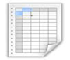Table 1Table 2Table 3